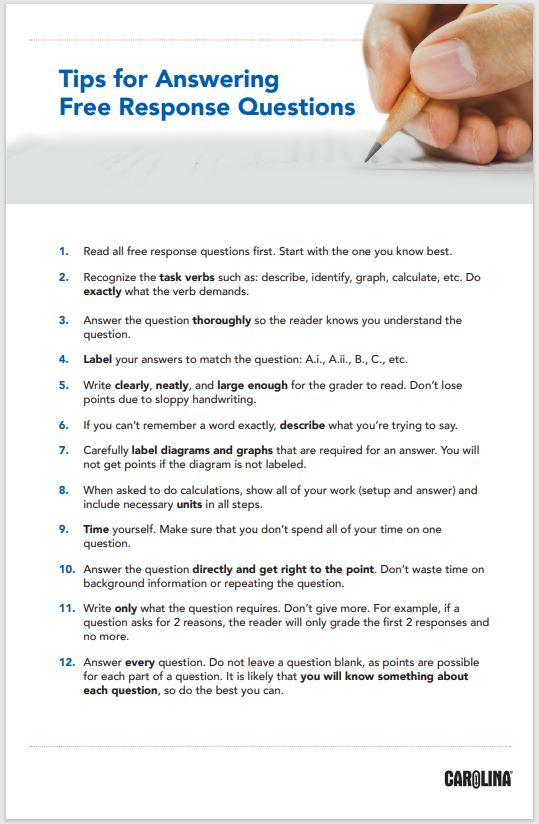# AP® Free-Response Questions: Dos and Don’ts## For all written responses

2. Pay close attention to verbs such as: describe, identify, discuss, graph, calculate, etc. Do exactly what the verb demands.
3. Write in complete sentences. Outlines, bullets, and diagrams will not be graded.
4. Your answer should be several sentences. Go into detail and answer the question thoroughly so the reader knows you understand the question.
5. Label your answers to match the question: A.i., A.ii., B., C., etc.
6. Write clearly, neatly, and large enough for the grader to read. Don’t lose points due to sloppy handwriting.
7. If you can’t remember a word exactly, describe what you are trying to say.
8. Use a pen with blue or black ink. Don’t use a pencil or a felt-tip pen.
9. Carefully label diagrams that are required for an answer. You will not get points if the diagram is not labeled.
10. When asked to do calculations, show all of your work (setup and answer), and include necessary units in all steps.
11. Time yourself. You have 90 minutes and 4 free response questions, so about 22 minutes per answer.
12.  Understand the exam is written on a college level. The national average for the free response section will be about 50% correct that is 5 points out of a possible 10 on each question. It is very likely that you will not know everything. However, it is very likely that you will know something about each question, so do the best you can.
13. Answer the question directly. Don’t waste time on background information or repeating the question.
14.  Get right to the point. Say what you know and go the next question. Don’t give an introductory paragraph just give the answer.
15. Write only what the question requires. Don’t give more. For example, if the question asked for 2 reasons, the reader will only grade the first 2 responses and no more.
16. Answer every question. Do not leave a question blank as points are possible for each part of the question.## Experimental design question

When asked to design an experiment:

1. Include a hypothesis and/or predictions.
2. Identify the independent variable. What treatment will you apply at what levels?
3. Identify the dependent variable. What will you measure?
4. Identify the control group and controlled variables (VERY IMPORTANT).
5. Describe the organism/materials/apparatus to be used.
6. Describe what you will actually do the procedure. (How will you apply the treatment?)
7. Describe how you will actually take and record data.
8. Describe how the data will be analyzed and visualized (graphed).
9. State how you will draw a conclusion. (Compare the results to the hypothesis and predictions.)
10. The experimental design needs to be at least theoretically possible.

## Graphing

Include the following information on all of your graphs:

1. Set up the graph with the independent variable on the x-axis and the dependent variable on the y-axis.
2. Label both axes with the variable name and unit.
3. Mark off axes in equal increments and label the hash marks.
4. Plot points and attempt to sketch a smooth, curved line or the line of best fit.
5. If more than one line is graphed on the same set of axes, label each curve. (Labeling is better than a legend.)
6. Give the graph a descriptive title.
Make an effort on every question.

## Don’t quit!

Flo Gullickson
College Board® Certified Consultant and AP® Exam Reader
AP® Environmental Science

Updated 2023